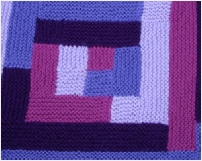﻿ Data LogBuy nowOther places to visit

Order Form

Mental Blocks

The World of Illusion Knittingfor this afghan

on the order form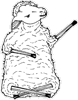Buy now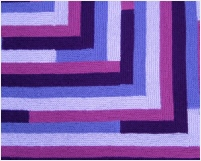DATA LOG

This afghan demonstrates where triangular numbers and square numbers coincide. A triangular number is the result of adding counting numbers. A square number is the result of multiplying a number by itself.

Example:

Triangular: 1 + 2 + 3 + 4 + 5 + 6 + 7 + 8 = 36

Square: 6 x 6 = 36

The answers in this example coincide.

The afghan shows where the 49th triangular number and 35th square number coincide, giving 1225 small squares.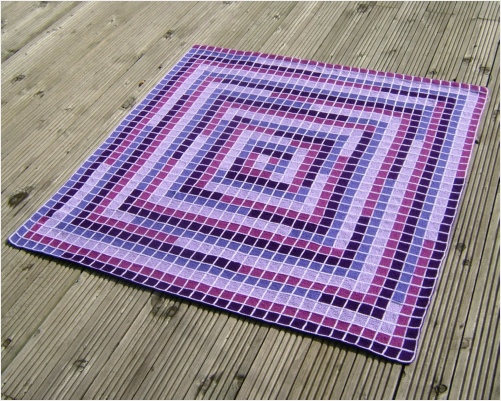RELATED DESIGNS

TRIANGULAR/SQUARE CUSHION

Tri-square

Double Vision

TRIANGULAR NUMBER AFGHANS

Try Angulate

Double VisionCONSTRUCTION INFORMATION

Knitted in one piece. The method is basically ‘log cabin’ although there are often two balls of yarn used in one stripe.

The optional grid is added with surface crochet, or embroidery, when the knitting is complete.KNITTING INFORMATION

The version in the photo was made using DK yarn. If you use a different type of yarn your afghan may be bigger, or smaller.

The pattern includes instructions for changing the overall size so you can use any yarn of your choice.

Data Log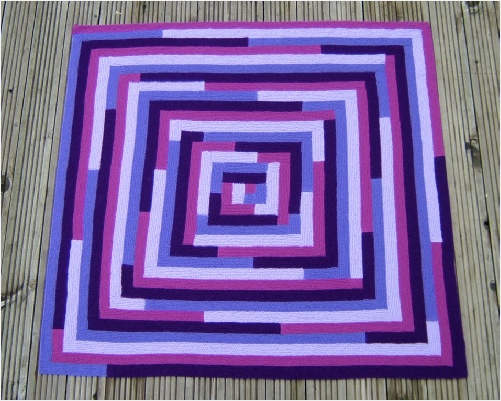The afghan can be made without the grid. The grid merely shows the mathematical idea more clearly as it makes it possible to count the number of squares in each stripe.

The next time triangular numbers and square numbers coincide is 41616. This would be a square with 204 squares along each side.

Click to see

larger pictures